## 探路者

5帖子
2回复
213积分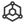ID:000045

2 2022-09-23

1. 首先需要确保北太天元已经加载了 绘图插件 graph， 可以在 帮助 》 插件 中查看，软件安装完成后会默认加载绘图插件。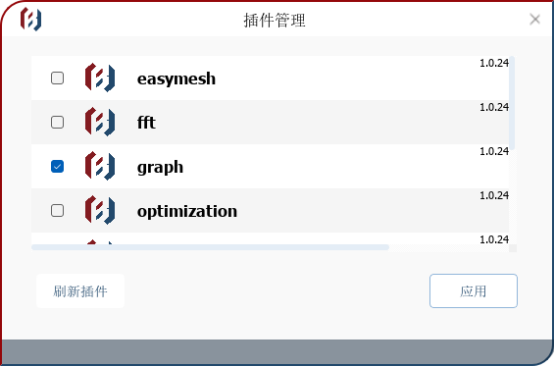2. 在绘图插件加载后，如何查看绘图插件提供哪些函数呢？有两个方法，一个是直接在命令行窗口输入 plugin_help(‘graph’) 就可以查看目前绘图插件提供的全部函数，或者在命令行窗口输入 help , 这个命令会返回北太天元里提供的所有内核提供的命令、脚本提供的命令和 插件 [graph(已授权)] 提供的命令。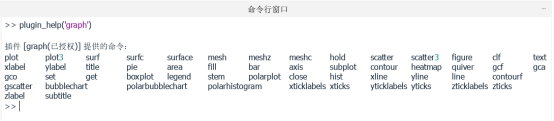3. 如何查看某个绘图函数的具体用法呢？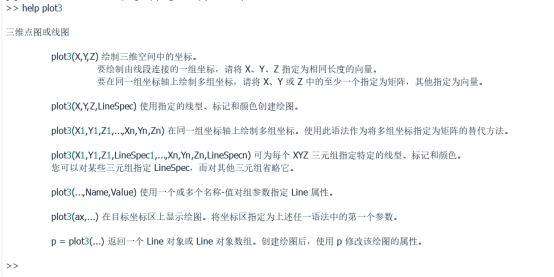t=0:pi/50:10*pi;

plot3(sin(t),cos(t),t);

axis square;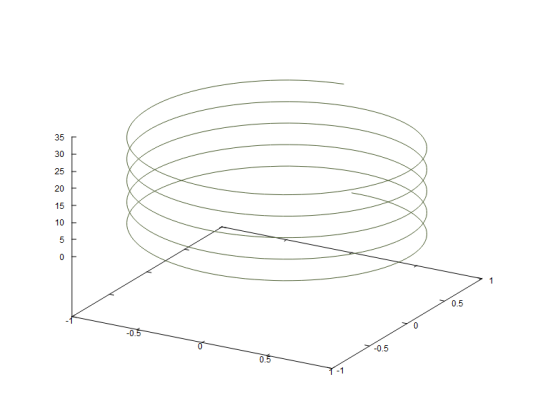### 下面介绍一下北太天元提供的二维图形

1.  条形图

bar(X,Y)：X是坐标，Y是高度，条形的跨度是x坐标的最小间距

bar(Y)：对Y绘制条形图。如果Y为矩阵，Y的每一行聚集在一起。横

2.  区域图

area(Y)：绘制向量Y。

area(X,Y)：绘制 Y 中的值对 x 坐标 X 的图。然后，该函数根据 Y 的形状填充曲线之间的区域：如果 Y 是向量，则该图包含一条曲线。area 填充该曲线和水平轴之间的区域。如果 Y 是矩阵，则该图对 Y 中的每列都包含一条曲线。area 填充这些曲线之间的区域并堆叠它们，从而显示在每个 x 坐标处每个行元素在总高度中的相对量。

3.  饼形图

pie(X)：使用 X 中的数据绘制饼图。饼图的每个扇区代表 X 中的一个元素。如果 sum(X) ≤ 1，X 中的值直接指定饼图扇区的面积。如果 sum(X) < 1，pie 仅绘制部分饼图。如果 sum(X) > 1，则 pie 通过 X/sum(X) 对值进行归一化，以确定饼图的每个扇区的面积。

4.  直方图

n=hist(Y)：绘制Y的直方图。

n=hist(Y,nbins)：指定分格的数目。

5.  针状图

stem(Y)：绘制Y的数据序列，图形起始于X轴，并在每个数据点处绘制一个小圆圈。

strm(X,Y)：按照指定的X绘制数据序列Y。

6.  方向矢量图和速度矢量图

quiver(x,y,u,v)：绘制矢量图，参数x和y用于指定矢量的位置，u和v用于指定要绘制的矢量。

quiver(u,v)：绘制矢量图，矢量的位置为默认值。

7.  等高线的绘制

contour 显示矩阵Z的二维等高线图

meshc 创建一个匹配有二维等高线图的网格图

contourf 显示矩阵Z的二维等高线图，并在各等高线之间用实体颜色填充

surfc 创建一个匹配有二维等高线图的曲面图

contour(Z)：绘制矩阵Z的等高线，绘制时将Z在x-y平面插值，等高线数量和数值由系统根据Z自动确定。

contour(X,Y,Z)：绘制矩阵Z的等高线，坐标值由矩阵X和Y指定，矩阵X、Y、Z的维数必须相同。

contour(X,Y,Z,“ShowText”,“on”)：绘制矩阵Z的等高线，坐标值由矩阵X和Y指定三维图形,通过ShowText后的参数为“on”或者“off”,设置图像是否显示标注。

### 三维图形

plot3(X,Y,Z)： 绘制三维空间中的坐标。要绘制由线段连接的一组坐标，请将 X、Y、Z 指定为相同长度的向量。要在同一组坐标轴上绘制多组坐标，请将 X、Y 或 Z 中的至少一个指定为矩阵，其他指定为向量。

plot3(X,Y,Z,LineSpec)： 使用指定的线型、标记和颜色创建绘图。

plot3(X1,Y1,Z1,...,Xn,Yn,Zn)： 在同一组坐标轴上绘制多组坐标。使用此语法作为将多组坐标指定为矩阵的替代方法。

plot3(X1,Y1,Z1,LineSpec1,...,Xn,Yn,Zn,LineSpecn)： 可为每个 XYZ 三元组指定特定的线型、标记和颜色。您可以对某些三元组指定 LineSpec，而对其他三元组省略它。

plot3(...,Name,Value)： 使用一个或多个名称-值对组参数指定 Line 属性。

1.  三维网格图

mesh函数用于绘制三维网格图，其调用语法如下。

mesh(X,Y,Z)： 创建一个网格图，该网格图为三维曲面，有实色边颜色，无面颜色。该函数将矩阵 Z 中的值绘制为由 X 和 Y 定义的 x-y 平面中的网格上方的高度。边颜色因 Z 指定的高度而异。

mesh(Z)： 创建一个网格图，并将 Z 中元素的列索引和行索引用作 x 坐标和 y坐标。

mesh(Z,C)： 进一步指定边的颜色。

mesh(___,C)： 进一步指定边的颜色。

mesh(ax,___)： 将图形绘制到 ax 指定的坐标区中，而不是当前坐标区中。指定坐标区作为第一个输入参数。

mesh(___,Name,Value)： 使用一个或多个名称-值对组参数指定曲面属性。例如，'FaceAlpha',0.5 创建半透明网格图。

2.  三维曲面图

surf(X,Y,Z) 创建一个三维曲面图，它是一个具有实色边和实色面的三维曲面。该函数将矩阵 Z 中的值绘制为由 X 和 Y 定义的 x-y 平面中的网格上方的高度。曲面的颜色根据 Z 指定的高度而变化。

surf(Z) 创建一个曲面图，并将 Z 中元素的列索引和行索引用作 x 坐标和 y 坐标。

0 2022-12-21
【春节篇】使用北太天元画灯笼

## 佳节临近，今天给大家分享一下如何使用北太天元绘制一个灯笼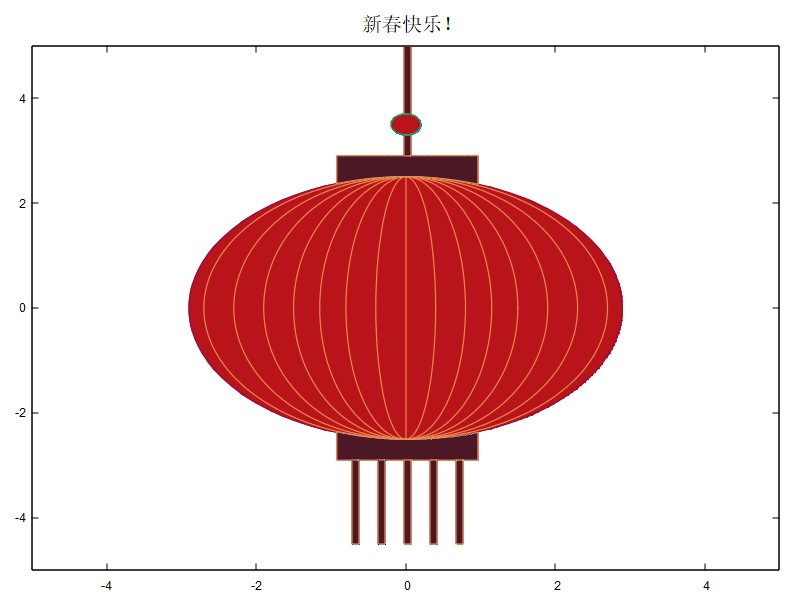```>> help fill

% 函数创建红色多边形。
% X 是顶点的x坐标，Y是顶点的y坐标
% 例如 一个三角形的三个顶点的坐标是p1(0,0), p2(1,0), p3(0.5,0.5)
% 画出红色的三角形
x = [0, 1, 0.5];
y = [0, 0, 0.5];
fill(x,y,'r')```

```% 椭圆数据点生成函数
function [X,Y]=getEllipse(Mu,XR,YR,theta,pntNum)
% Mu      |  中心点
% XR,YR   |  旋转前X,Y半轴长度
% theta   |  旋转角度
% pntNum |  生成数据点个数
tList = linspace(0,2*pi,pntNum);
X = cos(tList).*XR;
Y = sin(tList).*YR;
rotateMat = [cos(theta),-sin(theta);sin(theta),cos(theta)];
XY = rotateMat*[X;Y]+Mu(:);
X = XY(1,:);
Y = XY(2,:);
end```

```clf
hold on
n = 0.4;
x = 1;
a = 0.1;
w = 2.7;h = 2.5; %灯笼主体椭圆的宽和高

% 绘制灯笼主体
[X0,Y0]=getEllipse([0,0],2.9,h,0,200);
fill(X0,Y0,[184,20,25]./255,'EdgeColor',[153,12,40]./255,'LineWidth',1.2)

% 绘制辐线
[X,Y]=getEllipse([0,0],w,h,0,200);plot(X,Y,'Color',[236,136,74]./255,'LineWidth',1.2)
[X,Y]=getEllipse([0,0],w-n,h,0,200);plot(X,Y,'Color',[236,136,74]./255,'LineWidth',1.2)
[X,Y]=getEllipse([0,0],w-2*n,h,0,200);plot(X,Y,'Color',[236,136,74]./255,'LineWidth',1.2)
[X,Y]=getEllipse([0,0],w-3*n,h,0,200);plot(X,Y,'Color',[236,136,74]./255,'LineWidth',1.2)
[X,Y]=getEllipse([0,0],w-4*n+0.05,h,0,200);plot(X,Y,'Color',[236,136,74]./255,'LineWidth',1.2)
[X,Y]=getEllipse([0,0],w-5*n+0.1,h,0,200);plot(X,Y,'Color',[236,136,74]./255,'LineWidth',1.2)
[X,Y]=getEllipse([0,0],w-6*n+0.1,h,0,200);plot(X,Y,'Color',[236,136,74]./255,'LineWidth',1.2)
plot([0,0],[-h,h],'Color',[236,136,74]./255,'LineWidth',1.2)

% 计算其它部件需要的边界坐标
X1 = X0(abs(X0)<=x);
X2 = X1(1:size(X1,2)/2);
X3 = X1(size(X1,2)/2+1:end);
Y1 = Y0(abs(X0)<=x);
Y2 = Y1(1:size(Y1,2)/2);
Y3 = Y1(size(Y1,2)/2+1:end);
XX1 = X0(abs(X0)<=a);
XX2 = XX1(1:size(XX1,2)/2);
XX3 = XX1(size(XX1,2)/2+1:end);
YY1 = ones(1,size(XX2,2)).*(-w-0.2);
YY2 = ones(1,size(XX2,2)).*(-h-2.5);
YY3 = ones(1,size(XX2,2)).*(-h-2);

% 绘制其它部分
Y4 = ones(1,size(Y2,2)).*(w+0.2);
fill(X1,[Y2,Y4],[76,24,38]./255,'EdgeColor',[236,136,74]./255,'LineWidth',1.2)

Y5 = ones(1,size(Y2,2)).*-(w+0.2);
fill(X1,[Y3,Y5],[76,24,38]./255,'EdgeColor',[236,136,74]./255,'LineWidth',1.2)

fill(XX1,[YY1,YY3],[76,24,38]./255,'EdgeColor',[236,136,74]./255,'LineWidth',1.2)
fill(XX1.+0.7,[YY1,YY3],[76,24,38]./255,'EdgeColor',[236,136,74]./255,'LineWidth',1.2)
fill(XX1.-0.7,[YY1,YY3],[76,24,38]./255,'EdgeColor',[236,136,74]./255,'LineWidth',1.2)
fill(XX1.+0.35,[YY1,YY3],[76,24,38]./255,'EdgeColor',[236,136,74]./255,'LineWidth',1.2)
fill(XX1.-0.35,[YY1,YY3],[76,24,38]./255,'EdgeColor',[236,136,74]./255,'LineWidth',1.2)
fill(XX1,[-YY1,-YY2],[76,24,38]./255,'EdgeColor',[236,136,74]./255,'LineWidth',1.2)

[X6,Y6]=getEllipse([0,h+1],0.2,0.2,0,200);
fill(X6,Y6,[184,20,25]./255,'EdgeColor')

% 调整坐标轴
axis([-5 5 -5 5])
hold off
title("新春快乐！")```

0 2023-01-11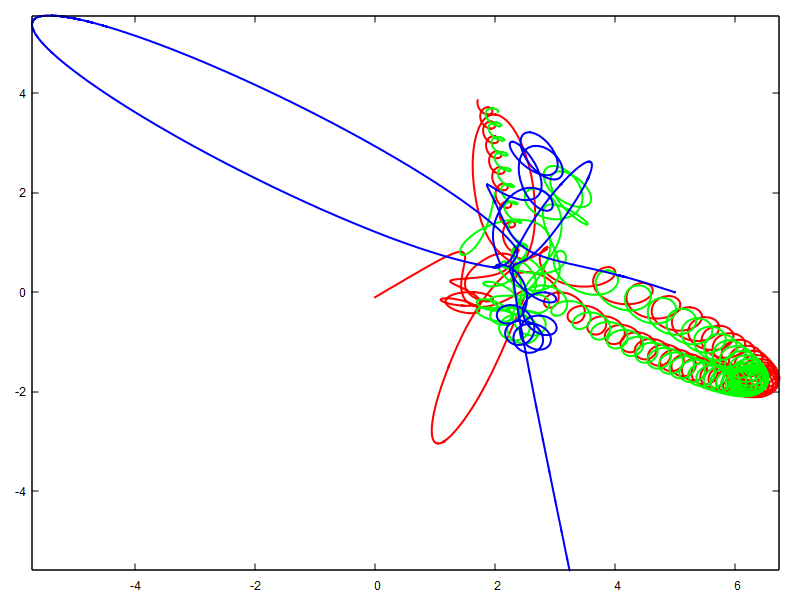（文章灵感来自卢朓老师的B站视频）

```三体（three-body problem）

----------------------------摘自百度百科```

```%模拟三个恒星组成的系统的三体运动
clear
%为了使用北太天元软件的pause插件函数
close all
% 三个恒星的质量都是1
ms = 1 ;
mt = 1 ;
mj = 1 ;
% 无量纲后万有引力常数设置为1
G = 1 ;
%初始条件 [xs,ys,xt,yt,xj,yj,vxs,vys,vxt,vyt,vjx,vjt]
CI = [0 -0.1 2 2 5 0 0 0 0 0 0 0];
%初始时刻to = 0;
%计算终止时刻t
f = 120;
%由位置的导数速度，速度的导数是加速，牛顿第二定律
% 以及万有引力定律得到常微分方程组
fxy = @(ps, pt, pj,ms,mt,mj) ...G*( mt.*(pt-ps)./norm(pt-ps).^3 ...+ mj.*(pj-ps)./norm(pj-ps).^3 );
F = @(t,Y) [Y(7);Y(8);Y(9);Y(10);Y(11);Y(12);...
fxy(Y([1,2]),Y([3,4]),Y([5,6]),ms,mt,mj); ...
fxy(Y([3,4]),Y([1,2]),Y([5,6]),mt,ms,mj); ...
fxy(Y([5,6]),Y([3,4]),Y([1,2]),mj,mt,ms); ...];
%使用ode45求解常微分方程组的初值问题
[t,Y]=ode45(F,[to,tf],CI);
%plot(Y(:,1),Y(:,2),'r',Y(:,3),Y(:,4),'g',Y(:,5),Y(:,6),'b')
yo = Y(1) ;
dto = 0.3 ;
plotmax = 100 ;
T=to ;
xmin = min(min(Y(:,[1,3,5])));
%三个质点的x坐标（在所有时刻)的最小值
xmax = max(max(Y(:,[1,3,5])));
ymin = min(min(Y(:,[2,4,6])));
%三个质点的y坐标（在所有时刻)的最小值
ymax = max(max(Y(:,[2,4,6])));
clf
close all
figure('Position',[0 0 1550 800])
hold off
told = 0;
for i = 1:length(Y(:,1))
dt = abs(Y(i,1)-yo)/abs(Y(i,7));
if dt >= dto
if i>plotmax
shift = plotmax;
else
shift = i-1;
end
plot(...
[xmin,xmax],[ymin,ymax], 'w', ... %画一个白色的斜线代替axis([xmin,xmax,ymin,ymax])设置画图范围
Y(i-shift:i,1),Y(i-shift:i,2),'r','LineWidth',2, ... %画第一个恒星在i-shift个时刻和第i个时刻件的轨迹
Y(i,1),Y(i,2),'-or','LineWidth',4, ... %画第一个恒星在第i个时刻所在的位置
Y(i-shift:i,3),Y(i-shift:i,4),'g','LineWidth',2, ...
Y(i,3),Y(i,4),'-og','LineWidth',4, ...
Y(i-shift:i,5),Y(i-shift:i,6),'b','LineWidth',2, ...
Y(i,5),Y(i,6),'-ob','LineWidth',4)
title(sprintf('时间=%f',t(i)))
T=[T;t(i)];
yo = Y(i,1) ;
vo = Y(i,7) ;
end
pause(0.01)
end
X=[0:1:length(T)-1];
figure(2)
plot(X,T)
plot(Y(:,1),Y(:,2),'r', 'LineWidth',2, ...
Y(:,3),Y(:,4),'g','LineWidth',2, ...
Y(:,5),Y(:,6),'b', 'LineWidth',2)

0 2023-02-10
Windows下使用MSYS2开发和SDK教程

### 首先是一些相关资源的分享：

1. Windows下MSYS2 开发环境的地址：打包好的MSYS2的百度云地址

2. SDK相关开发教程和视频： 开发示例文档 ， 开发教程视频

## 下面是如何在MSYS2 下编译SDK：

1. ### 项目的目录结构可以参考下面：

```myproject:
- include
- bin
- include
- lib
- src
- main.cpp
- *.cpp
- CMakeLists.txt```

### 2. SDK项目一般使用CMAKE 来进行编译的， 下面就是一个CMAKE需要的 CMakeLists.txt 的示例模板

```cmake_minimum_required(VERSION 3.10)
project(main VERSION 1.0.0)
# 将所有 .cpp 文件列出来
file(GLOB PLUGIN_SOURCE src/*.cpp)

# 由于是使用 dlopen() 载入，需要是 MODULE 类型
# 插件的文件名总是 main
set_target_properties(\${PROJECT_NAME} PROPERTIES OUTPUT_NAME "main")
# module 类型的库不需要 lib 前缀
set_target_properties(\${PROJECT_NAME} PROPERTIES PREFIX "")

# 链接 bex
set_target_properties(bex PROPERTIES INTERFACE_INCLUDE_DIRECTORIES \${PROJECT_SOURCE_DIR}/include/include)
set_target_properties(bex PROPERTIES IMPORTED_LOCATION \${PROJECT_SOURCE_DIR}/include/bin/libbex.dll)
set_target_properties(bex PROPERTIES IMPORTED_IMPLIB \${PROJECT_SOURCE_DIR}/include/lib/libbex.dll.a)

### 3. 如何使用 msys2 构建

```cd <my_project>
mkdir build && cd build```

`cmake .. -G "MinGW Makefiles"`

`nano ~/.bashrc`

`alias make='mingw32-make'`

`. ~/.bashrc`

`make -jN # N 表示开启的线程数`

`帮助 -> 插件`

---------------------------------------------------------------

0 2023-03-21